• 常用的dos功能调用指令 格式 作用 举例 键盘输入一个字符并显示(1号功能) INT 21H 按下任何键，将其对应字符ASCII码送入AL中，并在屏幕上显示该字符。如果按下是Ctrl＋Break组合键，则...

常用的dos功能调用：
指令
格式
作用
举例
键盘输入一个字符并显示(1号功能)

INT 21H

按下任何键，将其对应字符的ASCII码送入AL中，并在屏幕上显示该字符。如果按下的是Ctrl＋Break组合键，则终止程序执行。1号功能调用无须入口参数，出口参数在AL中

MOV AH,01H
INT 21H

键盘输入一个字符但不显示(8号功能)

MOV　AH,08H
INT 21H

同1号功能调用，但字符不在屏幕上显示。

MOV　AH,08H
INT 21H

屏幕显示一个字符(2号功能)

MOV DL,'字符’
MOV　AH,02H
ITN 21H

将置入DL寄存器中的字符在屏幕上显示输出。

MOV DL,'D'
MOV　AH,02H
INT 21H

打印输出(5号功能)

MOV DL,'字符'
MOV AH,05H
INT 21H

将置入DL寄存器中的字符送打印机接口，打印输出。

MOV DL, ‘d’
MOV AH, 05H
INT 21H

显示一个字符串(9号功能)

MOV DX, 字符串的偏移地址
MOV AH, 09H
INT 21H

在屏幕上显示出DS：DX指出的缓冲区中字符串。
在使用9号功能调用时，应当注意以下问题：
① 待显示的字符串必须先放在内存一数据区（DS段）中，且以‘$’符号作为结束标志。 ② 应当将字符串首地址的段基址和偏移地址分别存入DS和DX寄存器中。 字符串输入功能调用（0AH号功能调用） MOV DX, 已定义缓冲区的偏移地址 MOV AH, 0AH INT 21H 从键盘接收字符，并存放到内存缓冲区。 在使用0AH号功能调用时，应当注意以下问题： ① 执行前先定义一个输入缓冲区，缓冲区内第一个字节定义为允许最多输入的字符个数，字符个数应包括回车符0DH在内，不能为“0”值。第二个字节保留，在执 行程序完毕后存入输入的实际字符个数。从第三个字节开始存入从键盘上接收字符的ASCII码。若实际输入的字符个数少于定义的 ② 应当将缓冲区首地址的段基址和偏移地址分别存入DS和DX寄存器中。 返回DOS操作系统（4CH号功能调用） MOV AH, 4CH INT 21H 终止当前程序的运行，并把控制权交给调用的程序，即返回DOS系统，屏幕出现DOS提示符，如“C: \ >”，等待DOS命令。 直接输入、输出单字符（6号功能调用） MOV DL, 输入/输出标志 MOV AH, 06H INT 21H 执行键盘输入操作或屏幕显示输出操作，但不检查Ctrl＋Break组合键是否按下。执行这两种操作的选择由DL寄存器中的内容决定。 ① 当(DL)＝0FFH时，执行键盘输入操作。若标志ZF＝0，AL中放入字符的ASCII码；若标志ZF＝1，表示无键按下。这种调用用来检测键盘是否有键按下，但不等待键盘输入。 ② 当(DL)≠0FFH时，表示将DL中内容送屏幕显示输出。 检查键盘的工作状态（0BH号功能调用） MOV AH, 0BH INT 21H 检查是否有键盘输入，若有键按下，则使AL＝0FFH，若无键按下，则AL＝00H。对于利用键盘操作退出循环或使程序结束之类的操作来说，这种调用是很方便实用的。 设置系统日期（2BH号功能调用） MOV CX, 年号 MOV DH, 月号 MOV DL, 日期 MOV AH, 2BH INT 21H 设置有效的年、月、日。当AL＝0时，设置成功；当AL＝0FFH时，设置失败。 设置系统时间（2DH号功能调用） MOV CH, 小时 MOV CL, 分 MOV DH, 秒 MOV AH, 2DH INT 21H 设置有效的时间。当AL＝0时，设置成功；当AL＝0FFH时，设置失败。 转载于:https://www.cnblogs.com/asmer-stone/archive/2013/03/27/2983772.html 展开全文• ## 汇编dos功能调用 千次阅读 2017-10-18 21:52:45 第五版书上没有DOS指令以及BIOS指令，但是实验时候却要用到，特摘录如下。 一、 DOS软中断 DOS2.10使用了20H-27H,其功能定义为: 中断 功能 入口参数 出口参数 ...  第五版的书上没有DOS指令以及BIOS指令，但是实验的时候却要用到，特摘录如下。 一、 DOS软中断 DOS2.10使用了20H-27H,其功能定义为: 中断 功能 入口参数 出口参数 INT20 程序正常退出 CS=PSP段地址 INT21 系统功能调用 AH=功能号 INT22 程序结束处理 INT23 Ctrl-Break处理 AL=0(忽略) INT24 严重错误处理 AL=驱动器号 AL=1(重试) AL=2(通过INT 23H终止) Cy=1出错 INT25 绝对磁盘读 CX=读入扇区数 DX=起始逻辑扇区数 DS:BX=缓冲区地址 AL=驱动器号 Cy=0正确 INT26 绝对磁盘写 CX=写盘扇区数 DX=起始逻辑扇区数 DS:BX=缓冲区地址 INT27 驻留退出 CS=PSP段地址 DX=程序末地址+1 二、DOS功能调用 功能号在AH中,并设好其余的入口参数,向DOS发出INT21H命令,最后获得出口参数。 调用号 功能 入口参数 出口参数 00H 程序终止 CS=PSP段地址 01H 键盘输入字符 AL=输入的字符 02H 显示输出 DL=显示的字符 03H 串行设备输入 AL=输入的字符 04H 串行设备输出 DL=输出的字符 05H 打印输出 DL=输出的字符 06H 直接控制台I/O DL=0FFH(输入请求) DL=字符(输出请求) AL=输入的字符 07H 直接控制台I/O (不显示输入) AL=输入的字符 08H 键盘输入字符(无回显) AL=输入的字符 09H 显示字符串 DS:DX=缓冲区首址 0AH 输入字符串 DS:DX=缓冲区首址 0BH 检查标准输入状态 AL=00无按键 AL=0FFH有按键 0CH 清除输入缓冲区并执行指定的标准输入功能 AL=功能号 (01/06/07/08/0AH) DS:DX=缓冲区(0AH功能) AL=输入的数据 (功能01/06/07/08) 0DH 初始化磁盘状态 0EH 选择缺省的驱动器 DL=驱动器号(0=A,1=B..) AL=逻辑驱动器数 0FH 打开文件 DS:DX=未打开的FCB首址 AL=00成功,0FFH失败 10H 关闭文件 DS:DX=打开的FCB首址 AL=00成功,0FFH失败 11H 查找第一匹配目录 DS:DX=未打开的FCB首址 AL=00成功,0FFH失败 12H 查找下一匹配目录 DS:DX=未打开的FCB首址 AL=00成功,0FFH失败 13H 删除文件 DS:DX=未打开的FCB首址 AL=00成功,0FFH失败 14H 顺序读 DS:DX=打开的FCB首址 AL=00成功,01文件结束 02缓冲区太小 03缓冲区不满 15H 顺序写 DS:DX=打开的FCB首址 AL=00成功,01盘满 02缓冲区太小 16H 创建文件 DS:DX=未打开的FCB首址 AL=00成功 0FFH目录区满 17H 文件换名 DS:DX=被修改的FCB首址 AL=00成功,0FFH未找 到目录项或文件重名 *18H 保留未用 19H 取缺省驱动器号 AL=驱动器号(0=A,1=B..) 1AH 设置磁盘缓冲区DTA DS:DX=磁盘缓冲区首址 *1BH 取缺省驱动器的磁盘格 式信息 AL=每簇的扇区数 CX=每扇区的字节数 DX=数据区总簇数-1 DS:BX=介质描述字节 *1CH 取指定驱动器的磁盘格 式信息 DL=驱动器号(0=缺省, 1=A..) AL=每簇的扇区数 CX=每扇区的字节数 DX=数据区总簇数-1 DS:BX=介质描述字节 *1DH 保留未用 *1EH 保留未用 *1FH 取缺省驱动器的DPB DS:BX=DPB首址 *20H 保留未用 21H 随机读一个记录 DS:DX=打开的FCB首址 AL=00成功,01文件结束 02缓冲区太小 03缓冲区不满 22H 随机写一个记录 DS:DX=打开的FCB首址 AL=00成功,01盘满 02缓冲区太小 23H 取文件大小 DS:DX=未打开的FCB首址 AL=00成功,0FFH失败 24H 设置随机记录号 DS:DX=打开的FCB首址 25H 设置中断向量 AL=中断号 DS:DX=中断程序入口 *26H 创建新的PSP DS:DX=新的PSP段地址 27H 随机读若干记录 DS:DX=打开的FCB首址 CX=要读入的记录数 AL=00成功,01文件结束 AL=02缓冲区太小 AL=03缓冲区不满 CX=读入的块数 28H 随机写若干记录 DS:DX=打开的FCB首址 CX=要写入的记录数 AL=00成功,01盘满 AL=02缓冲区太小 AL=03缓冲区不满 CX=已写的块数 29H 分析文件名 AL=分析控制标记 DS:SI=要分析的字符串 ES:DI=未打开的FCB首址 AL=00未通配符 01有通配符 0FFH驱动器字母无效 ES:DI=未打开的FCB 2AH 取系统日期 CX=年(1980-2099) DH=月,DL=日,AL=星期 (0=星期日) 2BH 置系统日期 CX=年,DH=月,DL=日 AL=00成功,0FFH失败 2CH 取系统时间 CH=时(0-23),CL=分,DH= 秒,DL=百分之几秒 2DH 置系统时间 CX=时,分DX=秒,百分秒 AL=00成功,0FFH失败 2EH 设置/复位校验开关 AL=0关闭,1打开 2FH 取磁盘传输地址DTA ES:BX=DTA首地址 30H 取DOS版本 AL,AH=DOS主、次版本 31H 结束并驻留 AL=返回码,DX=内存大小 *32H 取指定驱动器的DPB DS:BX=DPB首址 33H 取或置Ctrl-Break标志 AL=0:取,1:置,DL=标志 DL=标志(取功能)0:关 1:开 *34H 取DOS中断标志 ES:BX=DOS中断标志 35H 取中断向量地址 AL=中断号 ES:BX=中断程序入口 36H 取磁盘的自由空间 DL=驱动器号(0=缺省,1=A AX=FF驱动器无效 其它每簇扇区数 BX=自由簇数 CX=每扇区字节数 BX=文件区所占簇数 *37H 取/置参数分隔符 取/置设备名许可标记 AL=0:取分隔符,1:置分隔 符,DL=分隔符 2:取许可标记 3:置许可标记,DL=许 可标记 DL=分隔符(功能0) DL=许可标记(功能2) 38H 取国家信息 AL=0,DS:DX=缓冲区首址 39H 创建子目录 DS:DX=路径字符串 CF=0成功,1失败,AX=错 误码 3AH 删除子目录 DS:DX=路径字符串 CF=0成功,1失败,AX=错 误码 3BH 设置子目录 DS:DX=路径字符串 CF=0成功,1失败,AX=错 误码 3CH 创建文件 DS:DX=带路径的文件名 CX=属性1-只读2-隐蔽 4-系统 CF=0成功,AX=文件号 CF=1失败,AX=错误码 3DH 打开文件 DS:DX=带路径的文件名 AL=方式0-读1-写2-读 写 CF=0成功,AX=文件号 CF=1失败,AX=错误码 3EH 关闭文件 BX=文件号 CF=0成功 CF=1失败,AX=错误码 3FH 读文件或设备 BX=文件号 CX=字节数 CF=0成功 DX:AX=新的指针位置 40H 写文件或设备 DS:DX=缓冲区首址 CF=1失败,AX=错误码 41H 删除文件 DS:DX=带路径的文件名 CF=0成功,1失败,AX=错 误码 42H 移动文件指针 AL=方式0-正向1-相对 2-反向 BX=文件号,CX:DX=移动的 位移量 CF=0成功,DX:AX=新的文 件指针 CF=1失败,AX=错误码 43H 取/置文件属性 AL=0:取1:置,CX=新属性 DS:DX=带路径的文件名 CX=属性(功能0)1-只读 2-隐蔽4-系统20H-归档 44H 设备输入/输出控制: 设置/取得与打开设备 的句柄相关联信息,或 发送/接收控制字符串 至设备句柄 AL=0/1取/置设备信息 2/3读/写设备控制通道 4/5同功能2/3 6/7取输入/输出状态 BX=句柄(功能0-3,6-7) BL=驱动器号(功能4-5) CX=字节数(功能2-5) DS:DX=缓冲区(功能2-5) CF=0成功 DX=设备信息(功能0) AL=状态(功能6/7) 0未准备,1准备 AX=传送的字节数(功能2- 5) 45H 复制文件号(对于一个 打开的文件返回一个新 的文件号) BX=文件号 CF=0成功,AX=新文件号 CF=1失败,AX=错误码 46H 强行复制文件号 BX=现存的文件号,CX=第2 文件号 CF=0成功,1失败 AX=错误码 47H 取当前目录 DL=驱动器号 DS:SI=缓冲区首址 CF=0成功,1失败 AX=错误码 48H 分配内存 BX=所需的内存节数 CF=0成功,AX=分配的段 数,CF=1失败,AX=错误码 BX=最大可用块大小 49H 释放内存 ES=释放块的段值 CF=1失败,AX=错误码 4AH 修改分配内存 ES=修改块的段值 BX=新长度(以节为单位) CF=1失败,AX=错误码 BX=最大可用块大小 4BH 装载程序 运行程序 AL=0装载并运行 1获得执行信息 3装载但不运行 DS:DX=带路径的文件名 ES:BX=装载用的参数块 CF=1失败,AX=错误码 4CH 带返回码的结束 AL=进程返回码 4DH 取由31H/4CH带回的返 回码 AL=进程返回码 AH=类型码,0-正常结束 1-由Ctrl-Break结束 2-由严重设备错误而结束 3-由调用31H而结束 4EH 查找第一个匹配项 DS:DX=带路径的文件名 CX=属性 CF=1失败,AX=错误码 4FH 查找下一个匹配项 CF=1失败,AX=错误码 *50H 建立当前的PSP段地址 BX=PSP段地址 *51H 读当前的PSP段地址 BX=PSP段地址 *52H 取DOS系统数据区首址 ES:BX=DOS数据区首址 *53H 为块设备建立DPB DS:SI=BPB,ES:DI=DPB 54H 取校验开关设定值 AL=标志值(0:关,1:开) *55H 由当前PSP建立新PSP DX=PSP段地址 56H 文件换名 DS:DX=带路径的旧文件名 ES:DI=带路径的新文件名 CF=1失败,AX=错误码 57H 取/置文件时间及日期 AL=0/1取/置,BX=文件号 CX=时间,DX=日期 CF=0成功,CX=时间,DX= 日期 CF=1失败,AX=错误码 错误码 错误类型 错误码 错误类型 01H 无效的功能号 0AH 不正确的环境 02H 文件未找到 0BH 不正确的格式 03H 路径未找到 0CH 无效的存取代码 04H 打开的文件太多 0DH 无效的数据 05H 拒绝存取 0EH 保留 06H 非法的文件号 0FH 指定的驱动器无效 07H 内存控制块破坏 10H 试图删除当前目录 08H 没有足够的内存空间 11H 非同一设备 09H 无效的内存块地址 12H 没有更多的文件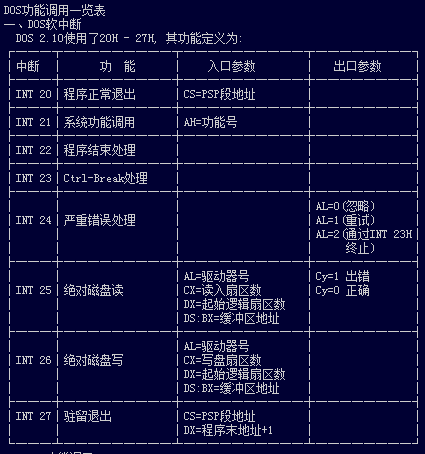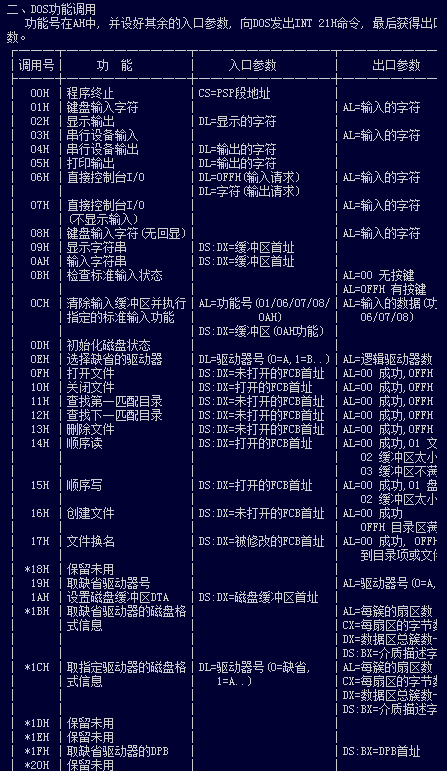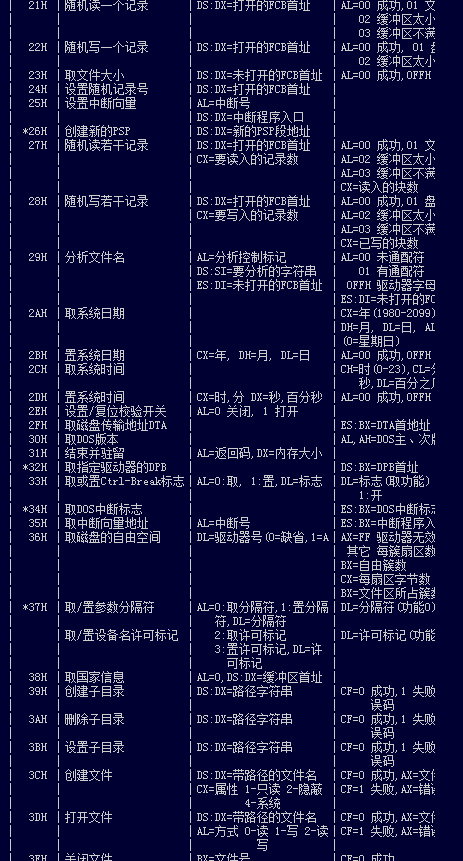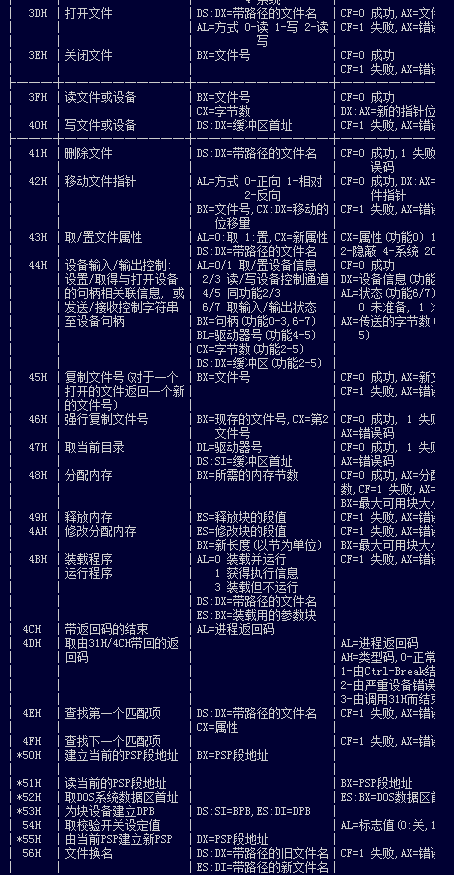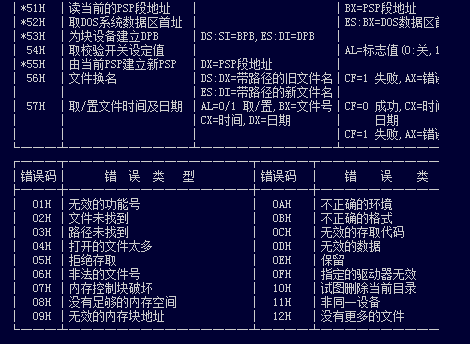展开全文• ## Dos功能调用 千次阅读 2013-01-05 23:16:54 子程序/系统功能调用包括入口参数，出口参数功能号和中断调用指令。 入口参数：传递数据（有些不需要） 出口参数：返回数据（有些子程序没有） 功能号： 子程序编号（必须送入AH寄存器） 中断调用... 子程序/系统功能调用包括入口参数，出口参数功能号和中断调用指令。 入口参数：传递的数据（有些不需要） 出口参数：返回的数据（有些子程序没有） 功能号： 子程序编号（必须送入AH寄存器） 中断调用：中断调用（INT 21H）展开全文• 很多初学汇编语言同学可能会对INT 21H这条指令感到困惑，不知道是什么意思，下面就以一段简单程序为大家讲解： 例如：需要键盘输入，并且回显。 AH值需要查表取得，表在下面 指令：MOV AH,01 INT 21H ... 转自：https://www.cnblogs.com/ynwlgh/archive/2011/12/12/2285017.html 很多初学汇编语言的同学可能会对INT 21H这条指令感到困惑，不知道是什么意思，下面就以一段简单的程序为大家讲解： 例如：需要键盘输入，并且回显。 AH的值需要查表取得，表在下面 指令：MOV AH,01 INT 21H 通过这样两条指令，输入的字符就会被存储在AL中。 表：DOS系统功能调INT 21H AH 功能 调用参数 返回参数 00 程序终止(同INT 20H) CS=程序段前缀 01 键盘输入并回显 AL=输入字符 02 显示输出 DL=输出字符 03 异步通迅输入 AL=输入数据 04 异步通迅输出 DL=输出数据 05 打印机输出 DL=输出字符 06 直接控制台I/O DL=FF(输入) DL=字符(输出) AL=输入字符 07 键盘输入(无回显) AL=输入字符 08 键盘输入(无回显) 检测Ctrl-Break AL=输入字符 09 显示字符串 DS:DX=串地址 '$'结束字符串

0A

键盘输入到缓冲区

DS:DX=缓冲区首地址
(DS:DX)=缓冲区最大字符数

(DS:DX+1)=实际输入的字符数

0B

检验键盘状态

AL=00 有输入
AL=FF 无输入

0C

清除输入缓冲区并
请求指定的输入功能

AL=输入功能号
(1,6,7,8,A)

0D

磁盘复位

清除文件缓冲区

0E

指定当前缺省的磁盘驱动器

DL=驱动器号 0=A,1=B,...

AL=驱动器数

0F

打开文件

DS:DX=FCB首地址

AL=00 文件找到
AL=FF 文件未找到

10

关闭文件

DS:DX=FCB首地址

AL=00 目录修改成功
AL=FF 目录中未找到文件

11

查找第一个目录项

DS:DX=FCB首地址

AL=00 找到
AL=FF 未找到

12

查找下一个目录项

DS:DX=FCB首地址
(文件中带有*或?)

AL=00 找到
AL=FF 未找到

13

删除文件

DS:DX=FCB首地址

AL=00 删除成功
AL=FF 未找到

14

顺序读

DS:DX=FCB首地址

AL=00 读成功
=01 文件结束,记录中无数据
=02 DTA空间不够
=03 文件结束,记录不完整

15

顺序写

DS:DX=FCB首地址

AL=00 写成功
=01 盘满
=02 DTA空间不够

16

建文件

DS:DX=FCB首地址

AL=00 建立成功
=FF 无磁盘空间

17

文件改名

DS:DX=FCB首地址
(DS:DX+1)=旧文件名
(DS:DX+17)=新文件名

AL=00 成功
AL=FF 未成功

19

取当前缺省磁盘驱动器

AL=缺省的驱动器号 0=A,1=B,2=C,...

1A

置DTA地址

DS:DX=DTA地址

1B

取缺省驱动器FAT信息

AL=每簇的扇区数
DS:BX=FAT标识字节
CX=物理扇区大小
DX=缺省驱动器的簇数

1C

取任一驱动器FAT信息

DL=驱动器号

同上

21

随机读

DS:DX=FCB首地址

AL=00 读成功
=01 文件结束
=02 缓冲区溢出
=03 缓冲区不满

22

随机写

DS:DX=FCB首地址

AL=00 写成功
=01 盘满
=02 缓冲区溢出

23

测定文件大小

DS:DX=FCB首地址

AL=00 成功(文件长度填入FCB)
AL=FF 未找到

24

设置随机记录号

DS:DX=FCB首地址

25

设置中断向量

DS:DX=中断向量
AL=中断类型号

26

建立程序段前缀

DX=新的程序段前缀

27

随机分块读

DS:DX=FCB首地址
CX=记录数

AL=00 读成功
=01 文件结束
=02 缓冲区太小,传输结束
=03 缓冲区不满

28

随机分块写

DS:DX=FCB首地址
CX=记录数

AL=00 写成功
=01 盘满
=02 缓冲区溢出

29

分析文件名

ES:DI=FCB首地址
DS:SI=ASCIIZ串
AL=控制分析标志

AL=00 标准文件
=01 多义文件
=02 非法盘符

2A

取日期

CX=年
DH:DL=月:日(二进制)

2B

设置日期

CX:DH:DL=年:月:日

AL=00 成功
=FF 无效

2C

取时间

CH:CL=时:分
DH:DL=秒:1/100秒

2D

设置时间

CH:CL=时:分
DH:DL=秒:1/100秒

AL=00 成功
=FF 无效

2E

置磁盘自动读写标志

AL=00 关闭标志
AL=01 打开标志

2F

取磁盘缓冲区的首址

ES:BX=缓冲区首址

30

取DOS版本号

AH=发行号,AL=版本

31

结束并驻留

AL=返回码
DX=驻留区大小

33

Ctrl-Break检测

AL=00 取状态
=01 置状态(DL)
DL=00 关闭检测
=01 打开检测

DL=00 关闭Ctrl-Break检测
=01 打开Ctrl-Break检测

35

取中断向量

AL=中断类型

ES:BX=中断向量

36

取空闲磁盘空间

DL=驱动器号
0=缺省,1=A,2=B,...

成功:AX=每簇扇区数
BX=有效簇数
CX=每扇区字节数
DX=总簇数
失败:AX=FFFF

38

置/取国家信息

DS:DX=信息区首地址

BX=国家码(国际电话前缀码)
AX=错误码

39

建立子目录(MKDIR)

DS:DX=ASCIIZ串地址

AX=错误码

3A

删除子目录（RMDIR）

DS:DX=ASCIIZ串地址

AX=错误码

3B

改变当前目录(CHDIR)

DS:DX=ASCIIZ串地址

AX=错误码

3C

建立文件

DS:DX=ASCIIZ串地址
CX=文件属性

成功:AX=文件代号
错误:AX=错误码

3D

打开文件

DS:DX=ASCIIZ串地址
AL=0 读
=1 写
=3 读/写

成功:AX=文件代号
错误:AX=错误码

3E

关闭文件

BX=文件代号

失败:AX=错误码

3F

读文件或设备

DS:DX=数据缓冲区地址
BX=文件代号
CX=读取的字节数

读成功:
AX=实际读入的字节数
AX=0 已到文件尾
读出错:AX=错误码

40

写文件或设备

DS:DX=数据缓冲区地址
BX=文件代号
CX=写入的字节数

写成功:
AX=实际写入的字节数
写出错:AX=错误码

41

删除文件

DS:DX=ASCIIZ串地址

成功:AX=00
出错:AX=错误码(2,5)

42

移动文件指针

BX=文件代号
CX:DX=位移量
AL=移动方式(0:从文件头绝对位移,1:从当前位置相对移动,2:从文件尾绝对位移)

成功:DX:AX=新文件指针位置
出错:AX=错误码

43

置/取文件属性

DS:DX=ASCIIZ串地址
AL=0 取文件属性
AL=1 置文件属性
CX=文件属性

成功:CX=文件属性
失败:CX=错误码

44

设备文件I/O控制

BX=文件代号
AL=0 取状态
=1 置状态DX
=2 读数据
=3 写数据
=6 取输入状态
=7 取输出状态

DX=设备信息

45

复制文件代号

BX=文件代号1

成功:AX=文件代号2
失败:AX=错误码

46

人工复制文件代号

BX=文件代号1
CX=文件代号2

失败:AX=错误码

47

取当前目录路径名

DL=驱动器号
DS:SI=ASCIIZ串地址

(DS:SI)=ASCIIZ串
失败:AX=出错码

48

分配内存空间

BX=申请内存容量

成功:AX=分配内存首地
失败:BX=最大可用内存

49

释放内容空间

ES=内存起始段地址

失败:AX=错误码

4A

调整已分配的存储块

ES=原内存起始地址
BX=再申请的容量

失败:BX=最大可用空间
AX=错误码

4B

装配/执行程序

DS:DX=ASCIIZ串地址
ES:BX=参数区首地址
AL=0 装入执行
AL=3 装入不执行

失败:AX=错误码

4C

带返回码结束

AL=返回码

4D

取返回代码

AX=返回代码

4E

查找第一个匹配文件

DS:DX=ASCIIZ串地址
CX=属性

AX=出错代码(02,18)

4F

查找下一个匹配文件

DS:DX=ASCIIZ串地址
(文件名中带有?或*)

AX=出错代码(18)

54

取盘自动读写标志

AL=当前标志值

56

文件改名

DS:DX=ASCIIZ串(旧)
ES:DI=ASCIIZ串(新)

AX=出错码(03,05,17)

57

置/取文件日期和时间

BX=文件代号
AL=0 读取
AL=1 设置(DX:CX)

DX:CX=日期和时间
失败:AX=错误码

58

取/置分配策略码

AL=0 取码
AL=1 置码(BX)

成功:AX=策略码
失败:AX=错误码

59

取扩充错误码

AX=扩充错误码
BH=错误类型
BL=建议的操作
CH=错误场所

5A

建立临时文件

CX=文件属性
DS:DX=ASCIIZ串地址

成功:AX=文件代号
失败:AX=错误码

5B

建立新文件

CX=文件属性
DS:DX=ASCIIZ串地址

成功:AX=文件代号
失败:AX=错误码

5C

控制文件存取

AL=00封锁
=01开启
BX=文件代号
CX:DX=文件位移
SI:DI=文件长度

失败:AX=错误码

62

取程序段前缀

BX=PSP地址


展开全文• 汇编之DOS系统功能调用 ...2.1 1号DOS功能调用 功能：单字符输入。 执行操作：将键盘输入字符ASCII码值送AL寄存器，同时回显在屏幕上。 入口参数：无 出口参数：AL寄存器存放输入字符ASCII码值 格式： MOV A
• （1）掌握简单DOS系统功能调用。 2、实验设备 微型计算机、emu8086 3、实验原理 本节要用到的DOS功能命令包括： （1）从键盘输入单个字符语句如下： MOV AH，1 INT 21H 以上两条指令执行后，（AL）=从键盘输入...编程语言
• 8086体系结构简介，80x86指令表，masm伪指令表，dos功能调用表，bios功能调用表。
• 汇编之控制转移指令之子程序、中断指令和系统功能调用前言子程序CALL调用指令RET返回指令中断指令和系统功能调用中断与中断源中断种类中断向量软中断指令中断响应过程中断嵌套中断处理程序设计设置中断向量DOS系统...
• 4.1 汇编语言中基本数据 ·标识符 ·常数 ·变量具有三个属性： （1）段地址（SEG）：变量所在段段地址 ...它可作为转移指令调用指令的目的操作数，以确定程序转移目的地址。 标号具有3个属性 （1...
• DOS功能调用 作者： 来源：互联网    MOV AH 4CH INT 21H 通过给AH寄存器赋值，然后调用INT 21H指令，计算机就会根据AH寄存器中值执行相应操作，下面是相应操作。  AH功能调用参数返回参数00 ...磁盘 c
• 这个汇编指令是用于提供DOS系统功能调用。它是由DOS提供一组实现特殊功能子程序供程序猿在编写自己程序时调用，以减轻编程工作量。 分两种，DOS系统功能调用和BIOS中断调用。 链接里都是一些经常使用...
• 文章目录第四章 汇编语言程序汇编语言程序结构汇编源程序的编程格式DOS调用BIOS功能调用键盘输入功能调用（INT 16H）BIOS屏显功能调用的调用模式（int 10H)程序设计方法汇编程序结构语法子程序设计宏指令程序设计 ...编程语言 linux 反汇编
• ## INT 21H 功能调用

千次阅读 2011-12-03 17:56:03
DOS的中断调用 比如 MOV AH 4CH INT 21H 是返回DOS系统 通过给AH寄存器赋值4CH，然后调用INT 21H指令，计算机就会根据AH...INT 21H 功能调用一览表 编号 功能 入口参数 出口参数 0 程序终止 CS=PSP磁盘
• 4.5.1 子程序：定义方法： 子程序名 PROC ...DOS功能调用：软中断指令 传送入口参数到指定寄存器中；MOV AH 4CH 调用的功能号送入AH寄存器中; INT 21H 4.5.3 一些功能号码 显示功能：02H(单字符...
• 目录控制转移类指令无条件转移指令JMP段内转移、直接寻址段内转移、间接寻址条件转移指令判断单个标志位状态比较无符号数高低比较有符号数大小循环指令(loop)子程序指令中断指令系统功能调用字符输出的功能调用字符...
• 本模块适合新手用来写一些dos命令，可以直接根据参数 dos指令，返回对应文本。跟系统自带的功能一样。但是非常方便调用和分析
• 一、返回DOS操作系统 返回DOS三种方法   程序框架设定...执行4CH号DOS功能调用。 MOV AX,4C00H INT 21H  利用这两条语句，在退出应用程序前，自动关闭已打开文件，防止数据丢失。 3. 对于可执行命令文
• 本程序主要是调用int 21h显示功能，可以用02h单个字符循环显示，也可以用09h显示字符串。 1.02h显示： DATA SEGMENT STR DB 'H', 'e', 'l', 'l', 'o', ',', 'L', 'C', ' ', 'U', 'n', 'i', 'v', 'e', '
• ## int指令

千次阅读 2012-09-19 15:35:03
因此，一般情况下，系统将一些具有一定功能的子程序，以中断处理程序方式提供给应用程序调用。 2、int和iret指令配合跟call和ret指令相似。 3、注意寄存器值保存和恢复。 4、对int、iret和栈深入理解：用7...byte c
• ## INT 21H 指令说明及使用方法

万次阅读 多人点赞 2018-04-13 11:35:56
很多初学汇编语言同学可能会对INT 21H这条指令感到困惑，不知道是什么意思，下面就以一段简单程序为...表：DOS系统功能调INT 21HAH功能调用参数返回参数00程序终止(同INT 20H)CS=程序段前缀　01键盘输入并回显　...
• dos中断的指令：int 中断处理程序入口程序CS:IP 中断号*4 例：int 21h 21h*4=84h (低字存放IP，高字存放CS) 则中断指令的入口地址 IP存放0084h，0085h 入口地址CS存放0086H 0087H 中断调用 INT 21H DOS中断...汇编语言
• Java执行Dos-Shell脚本1、介绍2、调用shell脚本2.1 获取键盘输入2.2 构建指令2.3 Java代码3、Java调用Shell并传入参数4、Java调用远程Shell脚本 相关参考内容原文地址： bldong:Java 执行Shell脚本指令 1、介绍 ...java linux shell
• MS-DOS一般使用命令行界面来接受用户的指令，不过在后期的MS-DOS版本中，DOS程序也可以通过调用相应的DOS中断来进入图形模式，即DOS下的图形界面程序。 早先版本的MS-DOS不支持FAT32、长文件名和大硬盘。从MS-DOS ...
• 通过这样两条指令，输入字符就会被存储在AL中。   表：DOS系统功能调INT 21H AH 功能 调用参数 返回参数 00 程序终止(同INT 20H) CS=程序段前缀 01 键盘输入并回显 AL=输入字符 02 显示输出 DL=输出...
• 保护 版权所有1995-1996 Philippe Paquet 描述 ProTecT是一种MS-DOS打包程序，...这允许使用没有可见代码结构的功能 返回指令可以是跳转或函数结尾不知道调用栈就不可能知道 反编译器将很难使用此技术 在不使用堆栈时
• 数据输入输出 希望能够直接从键盘上... 在汇编语言程序中，系统软件资源可以通过ROM BIOS中断调用和DOS系统功能调用中断来使用。前者对硬件依赖性强,可以更充分地驾驭硬件。后者使用更方便,但却无法实现某些功能。...